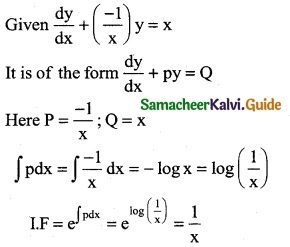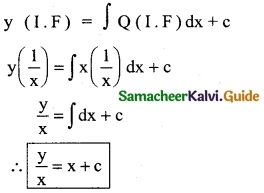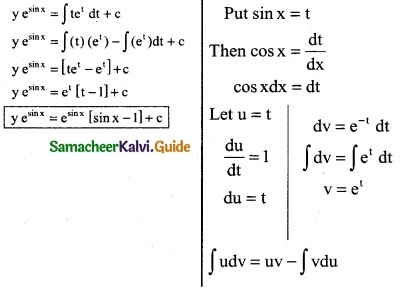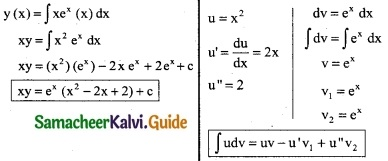Tamilnadu State Board New Syllabus Samacheer Kalvi 12th Business Maths Guide Pdf Chapter 4 Differential Equations Ex 4.4 Text Book Back Questions and Answers, Notes.

## Tamilnadu Samacheer Kalvi 12th Business Maths Solutions Chapter 4 Differential Equations Ex 4.4

Question 1.
$$\frac { dy }{dx}$$ – $$\frac { dy }{dx}$$ = x
Solution:The required solution isQuestion 2.
$$\frac { dy }{dx}$$ + y cos x = sin x cos x
Solution:
It is of the form $$\frac { dy }{dx}$$ + Py = Q
Here P = cos x; Q = sin x cos x
∫Pdx = ∫cos x dx = sin x
I.F = e∫pdx = esinx
The required solution is
Y(I.F) = ∫Q (IF) dx + c
Y(esinx) = ∫Q (I-F) dx + c
y (esinx) = ∫sin x cos x esinx dx + cQuestion 3.
x$$\frac { dy }{dx}$$ + 2y = x4
Solution:
The given equation can be reduced to
$$\frac { dy }{dx}$$ + $$\frac { 2y }{x}$$ = x³
It is of the form $$\frac { dy }{dx}$$ + Py = Q
Here P = $$\frac { 2 }{x}$$; Q = x³
∫pdx = ∫$$\frac { 2 }{x}$$dx = 2∫$$\frac { 1 }{x}$$dx = 2log x – log x²
I.F = e∫Pdx = elogx² = x²
The required solution is
y(I.F) = ∫Q (IF) dx + c
y(x²) = ∫x³ (x²) dx + c
x²y = ∫x5 dx + c
x²y = $$\frac { x^6 }{6}$$ + cQuestion 4.
$$\frac { dy }{dx}$$ + $$\frac { 3x^2 }{1+x^3}$$ = $$\frac { 1+x^2 }{1+x^3}$$
Solution:Question 5.
$$\frac { dy }{dx}$$ + $$\frac { y }{x}$$ = xex
Solution:
$$\frac { dy }{dx}$$ + py = Q
Here P = $$\frac { 1 }{x}$$; Q = xex
∫Pdx = ∫$$\frac { 1 }{x}$$ dx = log x
I.F = e∫Pdx = elog = x
The required solution is
y (I.F) = ∫Q (I.F) dx + cQuestion 6.
$$\frac { dy }{dx}$$ + y tan x = cos³ x
Solution:
It is of the form $$\frac { dy }{dx}$$ + Py = Q
Here P = tan x; Q = cos³ x
∫Pdx = ∫tan x dx = ∫$$\frac { sin x }{cos x}$$ dx = -∫$$\frac { -sin x }{cos x}$$ dx
= -log cos x = log sec x
I.F = e∫Pdx = elog sec x = sec x
The required solution is
y(I.F) = ∫Q(I.F) dx + c
y (sec x) = ∫cos³x (sec x) dx + c
y(sec x) = ∫cos³x $$\frac { 1 }{cos x}$$ dx + c
y (sec x) = ∫cos²x dx + c
y (sec x)= ∫($$\frac { 1+cos 2x }{2}$$) dx + c
y (sec x) = $$\frac { 1 }{2}$$ ∫(1 + cos2x) dx + c
y (sec x) = $$\frac { 1 }{2}$$ [x + $$\frac { sin2x }{2}$$] + cQuestion 7.
If $$\frac { dy }{dx}$$ + 2y tan x = sinx and if y = 0 when x = π/3 express y in terms of x
Solution:
$$\frac { dy }{dx}$$ + 2y tan x = sinx
It is of the form $$\frac { dy }{dx}$$ + Py = Q
Here P = 2tan x ; Q = sin x
∫Pdx = ∫2 tan x dx = 2∫tan xdx = 2 log sec x
log sec² x
I.F = e∫Pdx = elog(sec²x) = sec² x
The required solution is
y(I.F) = ∫Q(I.F) dx + c
y (sec² x) = ∫sin x (sec²x) dx + c
y(sec²x) = ∫sin x($$\frac { 1 }{cos x}$$) sec x dx + c
y sec²x = ∫($$\frac { sin x }{cos x}$$) sec x dx + c
y(sec²x) = ∫tan x sec x dx + c
⇒ y(sec²x) = sec x + c ………. (1)
If y = 0 when x = /3, then (1) ⇒
0(sec²(π/3)) = sec(π/3) + c
0 = 2 + c
⇒ c = -2
∴ Eqn (1) ⇒ y sec²x = sec x – 2

Question 8.
$$\frac { dy }{dx}$$ + $$\frac { y }{x}$$ = xex
Solution:
It is of the form $$\frac { dy }{dx}$$ + Py = Q
Here P = $$\frac { 1 }{x}$$; Q = xex
∫Pdx = ∫$$\frac { 1 }{x}$$ dx = log x
I.F = e∫Pdx = elog x = x
The required solution isQuestion 9.
A bank pays interest by contionous compounding, that is by treating the interest rate as the instantaneous rate of change of principal. A man invests Rs 1,00,000 in the bank deposit which accures interest, 8% over year compounded continuously. How much will he get after 10 years?
Solution :
Let P(t) denotes the amount of money in the account at time t. Then the differential equation govemning the growth of money is
$$\frac { dp }{dt}$$ = $$\frac { 8 }{100}$$p = 0.08 p
⇒ $$\frac { dp }{p}$$ = 0.08 dt
Integrating on both sides
∫$$\frac { dp }{p}$$ = ∫0.08 dt
loge P = 0.08 t + c
P = e0.08 t + c
P = e0.08 t. ec
P = C1 e0.08 t ………. (1)
when t = 0, P = Rs 1,00,000
Eqn (1) ⇒ 1,00,000 = C1
C1 = 1,00,000
∴ P = 100000 e0.08 t
At t = 10
P= 1,00,000 . e0.08(10)
= 1,00,000 e0.8 {∵ e0.8 = 2.2255}
= 100000 (2.2255)
p = Rs 2,25,550